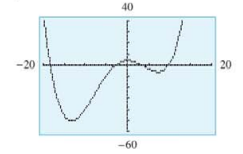Chapter 10, Problem 26RE### Mathematical Applications for the ...

11th Edition
Ronald J. Harshbarger + 1 other
ISBN: 9781305108042

#### Solutions

Chapter
Section### Mathematical Applications for the ...

11th Edition
Ronald J. Harshbarger + 1 other
ISBN: 9781305108042
Textbook Problem

# In Problems 25 and 26, a function and its graph are given.(a) Use the graph to determine (estimate) x-values where f ' ( x ) > 0, where f ’ ( x ) < 0, and where f ' ( x ) = 0.(b) Use the graph to determine x-values where f " ( x ) > 0, where f " ( x ) < 0, and where f " ( x ) = 0.(c) Check your conclusions to (a) by finding f ' ( x ) and graphing it with a graphing calculator.(d) Check your conclusions to (b) by finding f " ( x ) and graphing it with a graphing calculator. f ( x ) = 0.0025 x 4 + 0.02 x 3 − 0.48 x 2 + 0.08 x + 4(a)

To determine

The values of x for which f(x)>0,f(x)<0 and f(x)=0 from the graph of the function y=0.0025x4+0.02x30.48x2+0.08x+4.

Explanation

Given Information:

The provided function is y=0.0025x4+0.02x30.48x2+0.08x+4 and the graph is:

Explanation:

Consider the provided function, f(x)=0.0025x4+0.02x30.48x2+0.08x+4.

Use the simple power rule to differentiate the provided function,

ddx[f(x)]=ddx(0.0025x4+0.02x30.48x2+0.08x+4)f(x)=0.01x3+0.06x20.96x+0.08

The values of f(x) is zero when there is a turning point in the graph of f(x)=0.0025x4+0.02x30.48x2+0.08x+4.

The value of f(x) is positive when the function f(x)=0.0025x4+0.02x30.48x2+0.08x+4 increases as the value of x increases. That means the graph of the provided function has increasing nature.

Similarly, the value of f(x) is negative when the function f(x)=0.0025x4+0

(b)

To determine

The values of x for which f(x)>0,f(x)<0 and f(x)=0 from the graph of the function y=0.0025x4+0.02x30.48x2+0.08x+4.

(c)

To determine

Whether the conclusions made in part (a) are correct by graphing the derivative of the provided function f(x)=0.0025x4+0.02x30.48x2+0.08x+4.

(d)

To determine

Whether the conclusions made in part (b) are correct by graphing the second derivative of the provided function f(x)=0.0025x4+0.02x30.48x2+0.08x+4.

### Still sussing out bartleby?

Check out a sample textbook solution.

See a sample solution

#### The Solution to Your Study Problems

Bartleby provides explanations to thousands of textbook problems written by our experts, many with advanced degrees!

Get Started